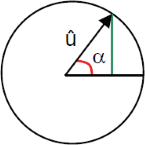# Voltage at a specific point in time

Calculating of the instantaneous voltage at a specific point in time

## Calculate instantaneous voltage

On this page you can calculate the instantaneous value of a sinusoidal oscillation at a specific point in time.

The reference voltage can be entered as an effective value or as a peak voltage.

Instantaneous voltage calculator

 Input Effective voltage Peak voltage V Frequency Hz kHz MHz GHz Time nSec µSec mSec Sec Decimal places 0 1 2 3 4 6 8 10 Result Effective voltage RMS Peak voltage Momentary voltage

## Formula for calculating the instantaneous value

With the help of the angular frequency formulas below, the instantaneous value of voltage and current can be determined after a certain time $$\displaystyle t$$.α 0° u = 0V α 90° u = û α 180° u = 0V α 270° u = û α 360° u = 0V

The pocket calculator must be set to radians to calculate these formulas.

Angular frequency $$\displaystyle ω= (2 · π · f)$$

$$\displaystyle u=û · sin(ω · t)$$   $$\displaystyle =û · sin(2 π f · t)$$

$$\displaystyle i=î · sin(ω · t)$$

#### Legend

 $$\displaystyle û$$ Peak voltage $$\displaystyle u$$ Instantaneous voltage $$\displaystyle f$$ Frequency $$\displaystyle t$$ Time $$\displaystyle ω$$ Angular frequency $$\displaystyle î$$ Peak current $$\displaystyle i$$ Instantaneous current

if you want to use degrees, you must convert the parameter to degree (x· 180/π)

Example:   $$\displaystyle u =û · sin\left(2 π f · t · \frac{180}{π}\right)$$